# Resample and Interpolate time series data

Resampling is a method of frequency conversion of time series data. You can use resample function to convert your data into the desired frequency.

Generally, the data is not always as good as we expect. For example: The data coming from a sensor is captured in irregular intervals because of latency or any other external factors

In order to work with a time series data the basic pre-requisite is that the data should be in a specific interval size like hourly, daily, monthly etc.

In this post we are going to explore the resample method and different ways to interpolate the missing values created by Downsampling or Upsampling of the data

## Let’s Get Started

### Load dataset to a Dataframe

This dataset contains 3 files of Timeseries data

it contains a datetime column and other columns are Temperature, Humidity, Light, CO2, HumidityRatio, Occupancy

Look at this data the dates are not in a specific interval. it’s just captured randomly.

By specific interval we meant the difference between the two successive date row should be something like 15 secs, 30 seconds, 30 minutes or 1 hour

``````import pandas as pd
df2 = pd.concat([df,df1])
df2[ 'date' ] = pd.to_datetime(df2['date'])
``````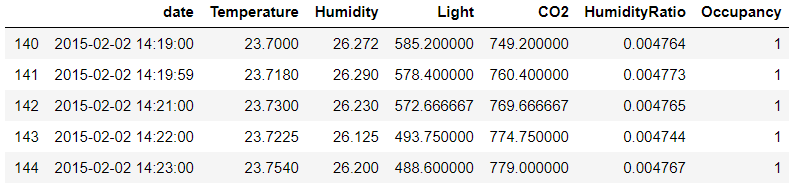## Downsample

For the resampling method we have to make sure the dataframe must have a datetime-like index (DatetimeIndex, PeriodIndex, or TimedeltaIndex), or pass datetime-like values to the on or level keyword

First we will set the date column as index using `set_index` function

The datetime columns should be a datetime object and not a string. if it is a string then convert to datetime using `pd.to_datetime()` method as we have done above

For using the resample() function we need to set the frequency for how we want to downsample or Upsample the timeseries data i.e. Hourly(H), Daily(D), 3 seconds(3s) etc.

We want to downsample and get the Hourly data so using ‘H’

Additionally, you have to also specify the function to apply on aggregated data. We have chosen a mean here

You can use your own custom function also on the resampler object that we will see in the following sections

``````# Resample Hourly
df3 = df2.set_index('date').resample('H').mean()
df3
``````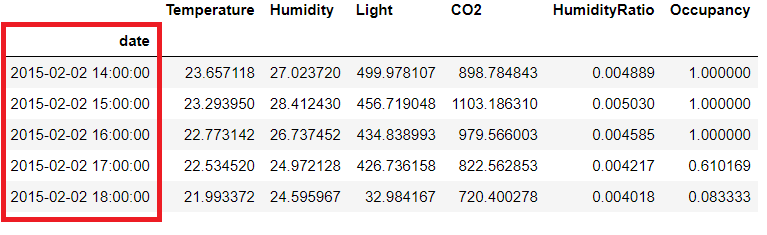## Upsample

In this section we will see how to upsample the timeseries data by increasing the frequency

In our original data we want to add more rows to see the datetime after every 3 seconds

``````# Resample 3 seconds
df2.set_index('date').resample('3s').mean()
``````

So here is the data after upsampling to 3 seconds with the mean for each of the column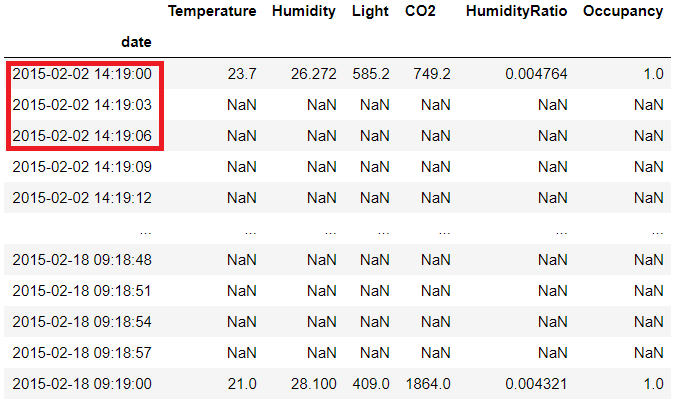You must be wandering from where those NaN values are coming

Since we don’t have original data for those timestamp so NaN is added by resample function

We will see in the Interpolation section below that how to fill those NaN values

## Apply Function

You can apply your own custom function to the aggregated data after resampling

In this example we are finding the difference between the max and min value for every hour in the original data

A lambda function is used here which is then passed to the pipe

``````# Apply func
df2.set_index('date').resample('H').pipe(lambda x: x.max() - x.min())

or

df2.set_index('date').resample('H').apply(lambda x: x.max() - x.min())
``````

## Offset

For example in this resampled function we are adding an offset value of 10 seconds

``````df2.set_index('date').resample('H',loffset='10s').mean()
``````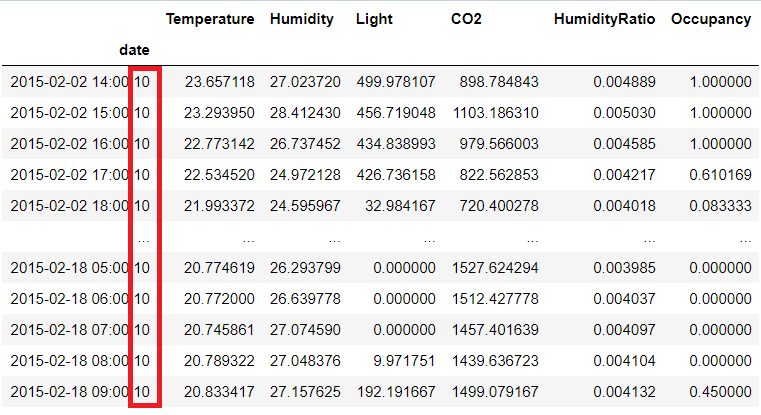## Interpolate

You can use interpolate function to fill those NaN rows created above after resampling using different methods like pad, Linear, quadratic, Polynomial, spline etc.

Let’s take the first example where we resampled the data hourly and check the number of rows with NaN values that are created during resampling

We will just check one column where the NaN values are created. So I will pick temperature here

``````df[df['Temperature'].isna()]
``````

So there are 171 rows which have NaN values which is created by resample function since there was no data available for these hours in the original data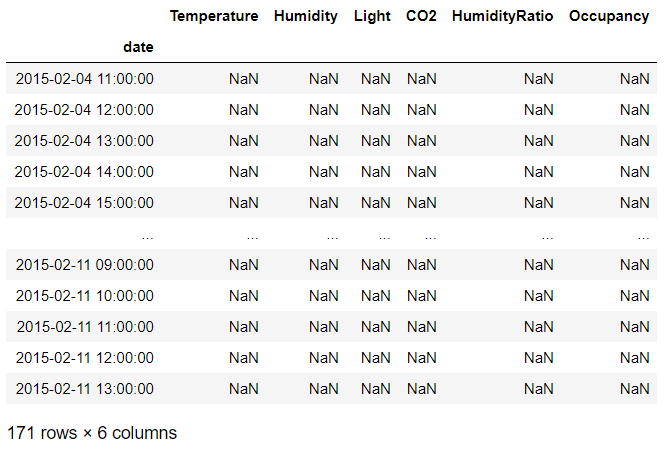I will plot this data after filling the nulls with zero for the time being

``````import seaborn as sns
sns.set(rc={'figure.figsize':(11,4)})
df3.fillna(0).plot()
``````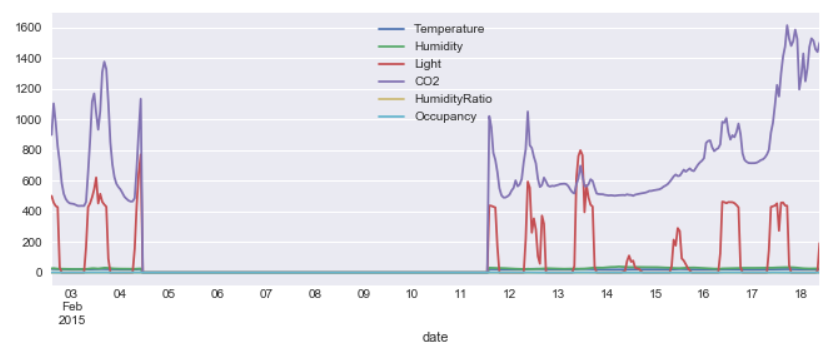Can you see that gap between 05 and 11 that is all the values which were NaN’s and filled by Zero for plotting

Now let’s understand how to fill the Null values(NaN) here with interpolate function

## Linear Interpolation:

As per wiki:

linear interpolation is a method of curve fitting using linear polynomials to construct new data points within the range of a discrete set of known data points

We are using temperature column (Series object) to fill the Nan’s and plot the data. You can use a dataframe object as well

# Linear Interpolate

``````df3['Temperature'].interpolate('linear').plot()
``````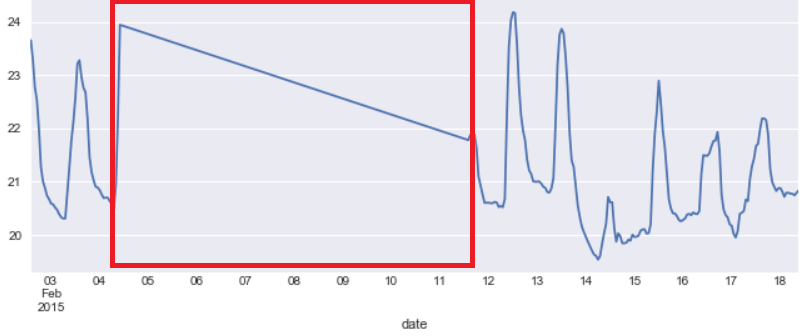To fill the NaN values by this method if we take two points i.e. one point before the NaN values and one point after the NaN value.

And draw a straight line between these two points then all the points fall on this line and that will be used for filling the NaN’s

This is evident from the figure above for Temperatue column. There is a linear line between date 05 and 11 where the original gap(NaN) in the data was found

Let’s check the values in dataframe after Linear Interpolation

``````df3 = df2.set_index('date').resample('H').mean().interpolate('linear')
df3[(df3.index > '2015-02-05')&(df3.index < '2015-02-11')]
``````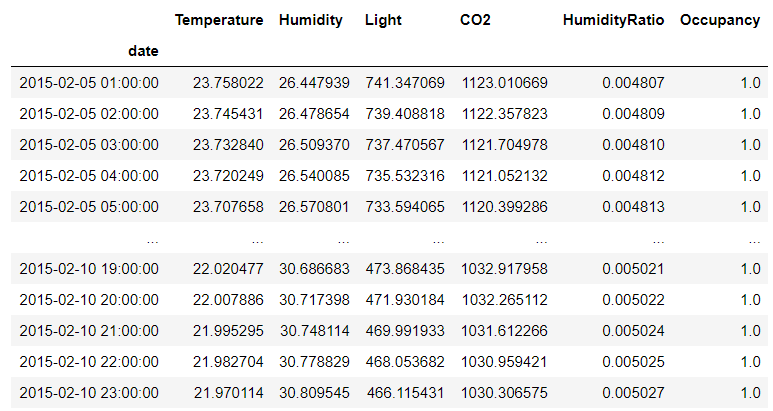## Polynomial Interpolation

With Polynomial interpolation method we are trying to fit a polynomial curve for those missing data points

There are different method of Polynomial interpolation like polynomial, spline available

You need to specify the order for this interpolation method

``````# Polynomial Interpolation
df3['Temperature'].interpolate('Polynomial', order=2).plot()
``````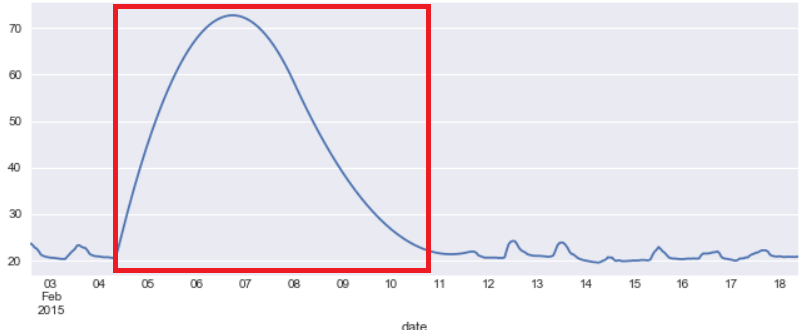Let’s see the real values in the dataframe now

``````df3 = df2.set_index('date').resample('H').mean().interpolate('polynomial',order=2)
df3[(df3.index > '2015-02-05')&(df3.index < '2015-02-11')]
``````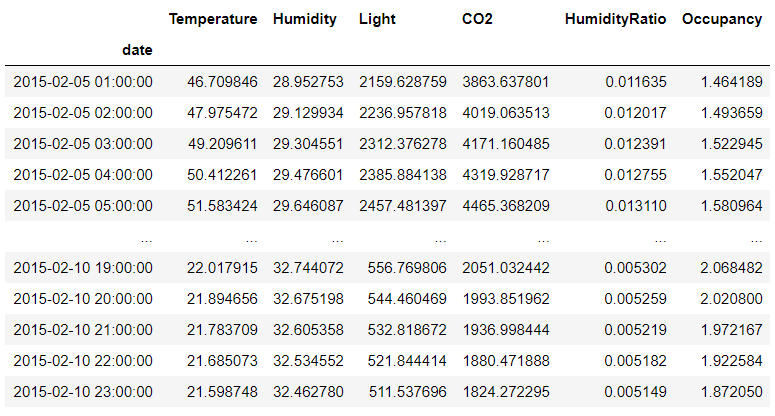First we resample the original dataframe to Hourly and applied mean

Next all the NaN values are filled using interpolate function using Polynomial interpolation of order 2

And finally filtering those values to get all the rows which were originally returned NaN by resample method for date 05 to 11

## Scipy Interpolate

For most of the interpolation methods  scipy.interpolate.interp1d is used in the background.

This class returns a function whose call method uses interpolation to find the value of new points.

Here are some of the interpolation methods which uses scipy backend

nearest, zero, slinear, quadratic, cubic, spline, barycentric, polynomial

You can create two arrays and interpolate will find the function between the two using the specified kind of interpolation

``````import numpy as np
from scipy import interpolate

x = np.arange(0,10)
y = np.exp(-x/10.0)
f = interpolate.interp1d(x, y,kind=5, fill_value='extrapolate')
``````

Now we can use function f to find y for any new value of x

## Conclusion

Here are the key points to summarize whatever we discussed in this post

1. How to resample timeseries data using pandas resample function using different frequency methods
2. Apply custom function to aggregated data after resampling
3. offset time data using loffset parameter
4. Interpolate the missing data using Linear and Polynomial Interpolation
5. Scipy Interpolation which is used as backend for the most interpolation methods in Pandas

Tags:

Categories:

Updated: